# Index Activity 1 Activity 2 Activity 3 Activity

• Slides: 31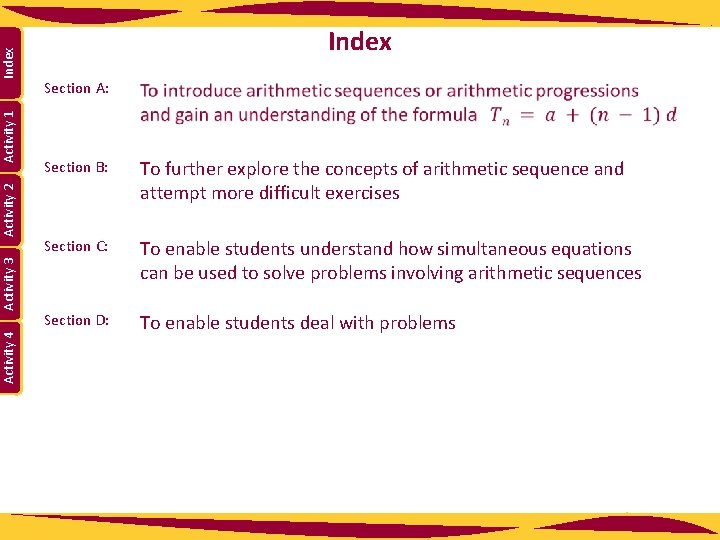Index Activity 1 Activity 2 Activity 3 Activity 4 Index Section A: Section B: To further explore the concepts of arithmetic sequence and attempt more difficult exercises Section C: To enable students understand how simultaneous equations can be used to solve problems involving arithmetic sequences Section D: To enable students deal with problems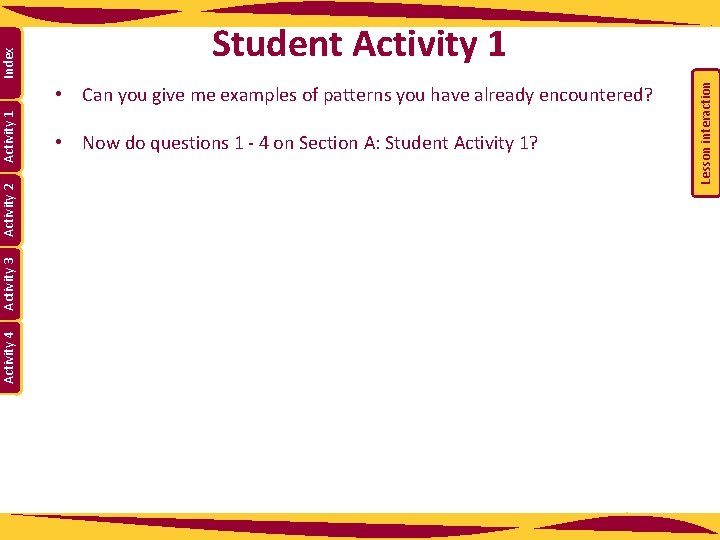• Can you give me examples of patterns you have already encountered? • Now do questions 1 - 4 on Section A: Student Activity 1? Lesson interaction Index Activity 1 Activity 2 Activity 3 Activity 4 Student Activity 1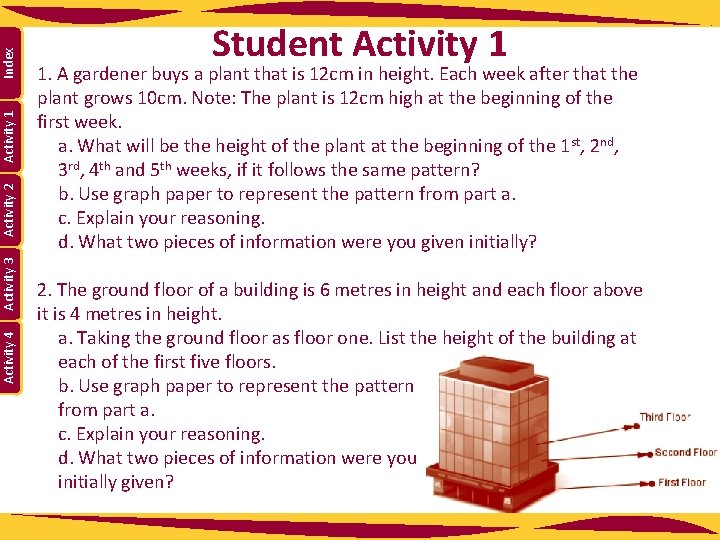Index Activity 1 Activity 2 Activity 3 Activity 4 Student Activity 1 1. A gardener buys a plant that is 12 cm in height. Each week after that the plant grows 10 cm. Note: The plant is 12 cm high at the beginning of the first week. a. What will be the height of the plant at the beginning of the 1 st, 2 nd, 3 rd, 4 th and 5 th weeks, if it follows the same pattern? b. Use graph paper to represent the pattern from part a. c. Explain your reasoning. d. What two pieces of information were you given initially? 2. The ground floor of a building is 6 metres in height and each floor above it is 4 metres in height. a. Taking the ground floor as floor one. List the height of the building at each of the first five floors. b. Use graph paper to represent the pattern from part a. c. Explain your reasoning. d. What two pieces of information were you initially given?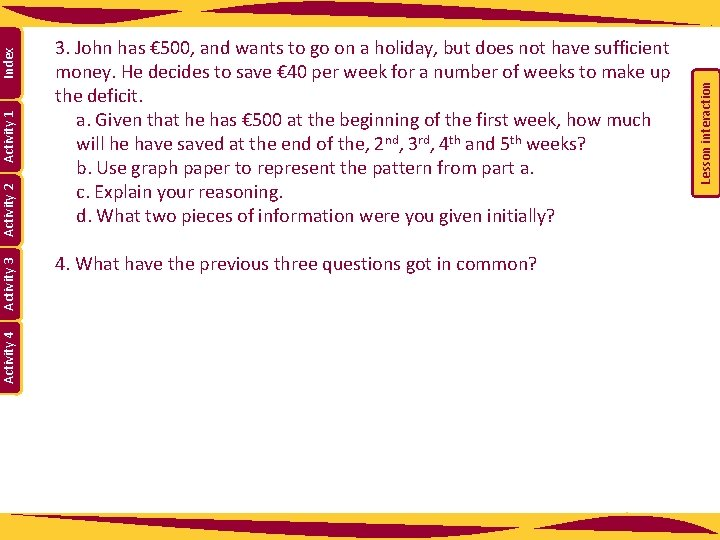4. What have the previous three questions got in common? Lesson interaction Index Activity 1 Activity 2 Activity 3 Activity 4 3. John has € 500, and wants to go on a holiday, but does not have sufficient money. He decides to save € 40 per week for a number of weeks to make up the deficit. a. Given that he has € 500 at the beginning of the first week, how much will he have saved at the end of the, 2 nd, 3 rd, 4 th and 5 th weeks? b. Use graph paper to represent the pattern from part a. c. Explain your reasoning. d. What two pieces of information were you given initially?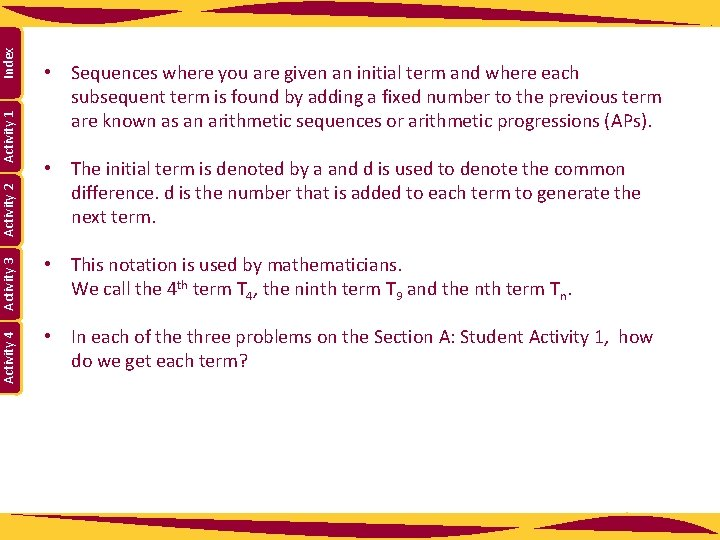Index Activity 1 Activity 2 Activity 3 Activity 4 • Sequences where you are given an initial term and where each subsequent term is found by adding a fixed number to the previous term are known as an arithmetic sequences or arithmetic progressions (APs). • The initial term is denoted by a and d is used to denote the common difference. d is the number that is added to each term to generate the next term. • This notation is used by mathematicians. We call the 4 th term T 4, the ninth term T 9 and the nth term Tn. • In each of the three problems on the Section A: Student Activity 1, how do we get each term?• Write out the next three terms. • Do you notice a pattern occurring? • So what is T 8? • What is T 38? • What is T 107? • What is Tn? Lesson interaction • Then move on to T 2. If we know what T 1 is, how do we find T 2? Lesson interaction Index Activity 1 Activity 2 Activity 3 Activity 4 Now let’s see if we can come up with a formula for T n? • Start with T 1. What is T 1 in terms of a and d?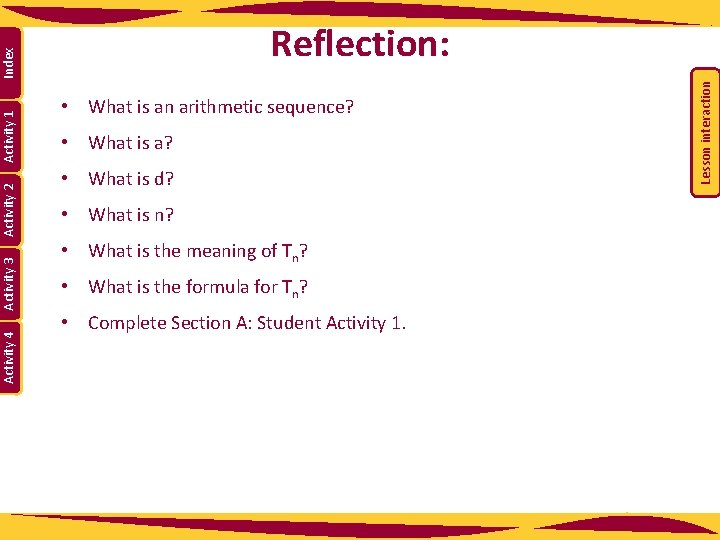Activity 4 Activity 3 • What is an arithmetic sequence? • What is a? • What is d? • What is n? • What is the meaning of Tn? • What is the formula for Tn? • Complete Section A: Student Activity 1. Lesson interaction Activity 2 Activity 1 Index Reflection:Index Activity 1 Activity 2 Activity 3 Activity 4 5. Find T 10 and T 12 for questions 1, 2 and 3 above. Formula for T 10 Question 1 Question 2 Question 3 Value for T 10 Formula for T 12 Value for T 127. Find T 1, T 2, T 3, T 5, T 10, and T 100 of an arithmetic sequence where a = 3 and d = -2. Index Activity 4 Activity 3 Activity 2 Activity 1 6. Find T 1, T 2, T 3, T 5, T 10, and T 100 of an arithmetic sequence where a = 4 and d = 6 8. Find T 1, T 2, T 3, T 5, T 10, and T 100 of an arithmetic progression where a = 3 and d = ½.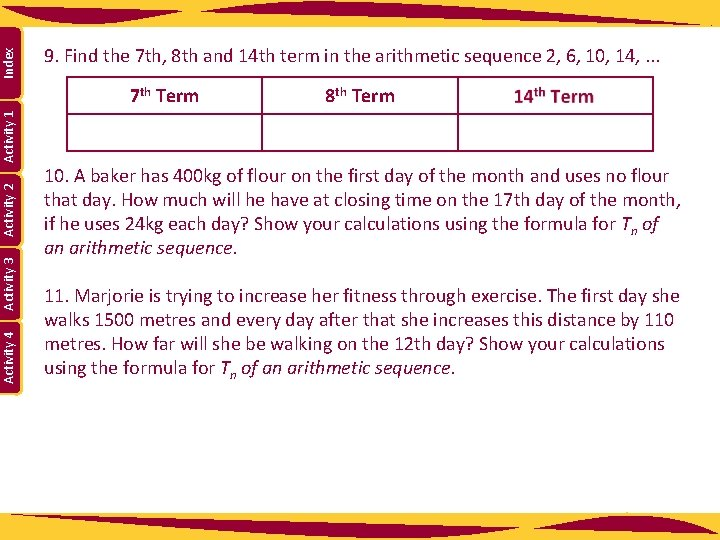7 th Term 8 th Term Activity 4 Activity 3 Activity 2 Activity 1 Index 9. Find the 7 th, 8 th and 14 th term in the arithmetic sequence 2, 6, 10, 14, . . . 10. A baker has 400 kg of flour on the first day of the month and uses no flour that day. How much will he have at closing time on the 17 th day of the month, if he uses 24 kg each day? Show your calculations using the formula for Tn of an arithmetic sequence. 11. Marjorie is trying to increase her fitness through exercise. The first day she walks 1500 metres and every day after that she increases this distance by 110 metres. How far will she be walking on the 12 th day? Show your calculations using the formula for Tn of an arithmetic sequence.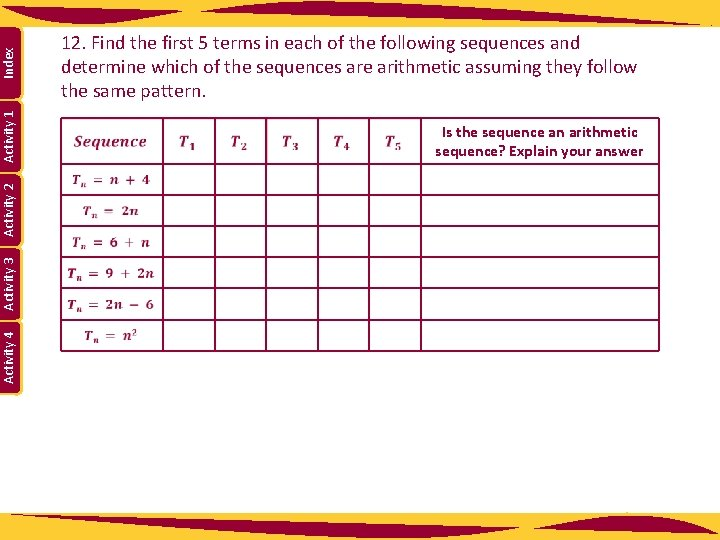Index Activity 1 Is the sequence an arithmetic sequence? Explain your answer Activity 4 Activity 3 Activity 2 12. Find the first 5 terms in each of the following sequences and determine which of the sequences are arithmetic assuming they follow the same pattern.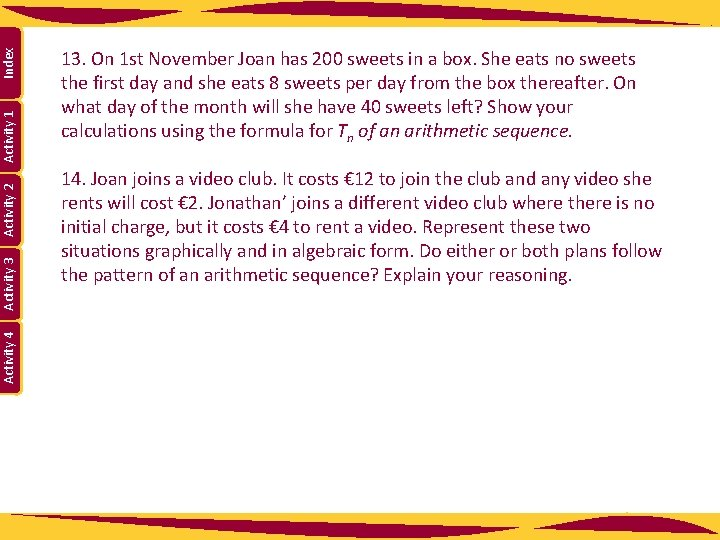Index Activity 1 Activity 2 Activity 3 Activity 4 13. On 1 st November Joan has 200 sweets in a box. She eats no sweets the first day and she eats 8 sweets per day from the box thereafter. On what day of the month will she have 40 sweets left? Show your calculations using the formula for Tn of an arithmetic sequence. 14. Joan joins a video club. It costs € 12 to join the club and any video she rents will cost € 2. Jonathan’ joins a different video club where there is no initial charge, but it costs € 4 to rent a video. Represent these two situations graphically and in algebraic form. Do either or both plans follow the pattern of an arithmetic sequence? Explain your reasoning.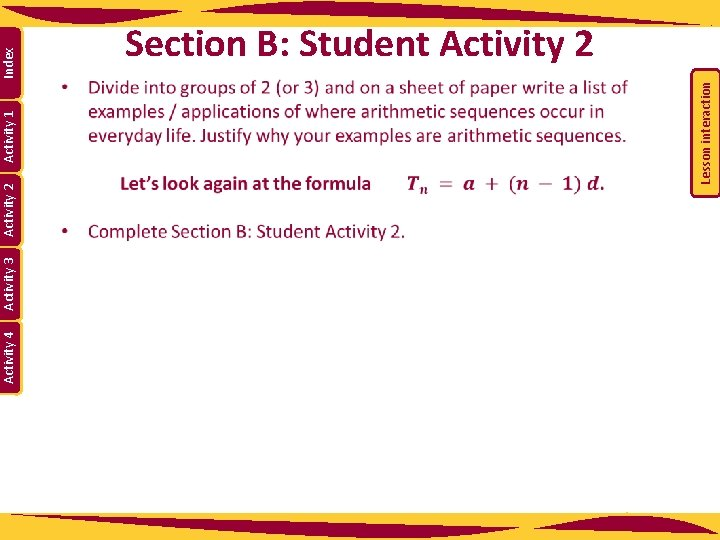Activity 4 Activity 3 Activity 1 Index Lesson interaction Activity 2 Section B: Student Activity 2Index Activity 1 Activity 2 Activity 3 Activity 4 Section B: Student Activity 2 1. An art collection is valued at € 50, 000 in 2001 and its value increases by € 4, 000 annually. a. Show that, as time passes, the value of the collection follows an arithmetic sequence? List the first ten terms of the sequence and give the first term and common difference. b. How much will the collection be worth in 2051? 2. A new car was valued at € 21, 000 on 1 st January 2001, and depreciated in value by a regular amount each year. On 1 st January 2008, it had a value of zero. Show that the value of the car could follow an arithmetic sequence. How many terms are there in this sequence? By how much did it depreciate each year? Show your calculations. 3. The sum of the interior angles of a triangle is 180°, of a quadrilateral is 360° and of a pentagon is 540°. Assuming this pattern continues, find the sum of the interior angles of a dodecagon (12 sides). Show your calculations. http: //www. regentsprep. org/Regents/math/algtrig/ATP 2/Sequence. Wordpractice. htmrow seat?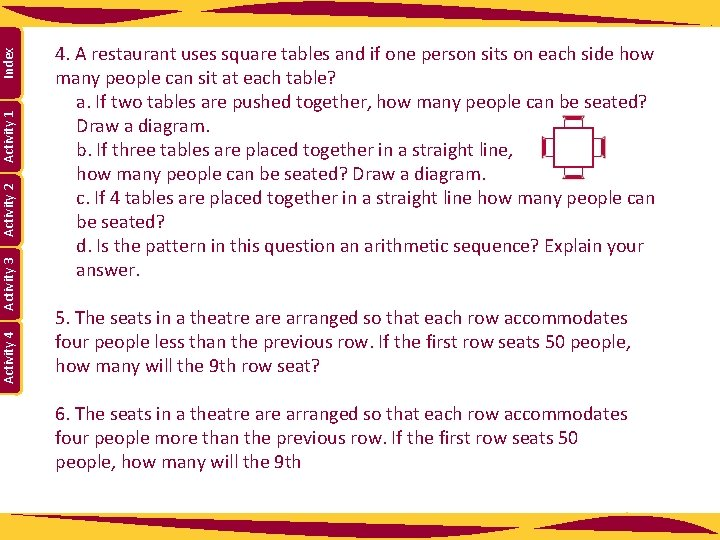Index Activity 1 Activity 2 Activity 3 Activity 4 4. A restaurant uses square tables and if one person sits on each side how many people can sit at each table? a. If two tables are pushed together, how many people can be seated? Draw a diagram. b. If three tables are placed together in a straight line, how many people can be seated? Draw a diagram. c. If 4 tables are placed together in a straight line how many people can be seated? d. Is the pattern in this question an arithmetic sequence? Explain your answer. 5. The seats in a theatre arranged so that each row accommodates four people less than the previous row. If the first row seats 50 people, how many will the 9 th row seat? 6. The seats in a theatre arranged so that each row accommodates four people more than the previous row. If the first row seats 50 people, how many will the 9 th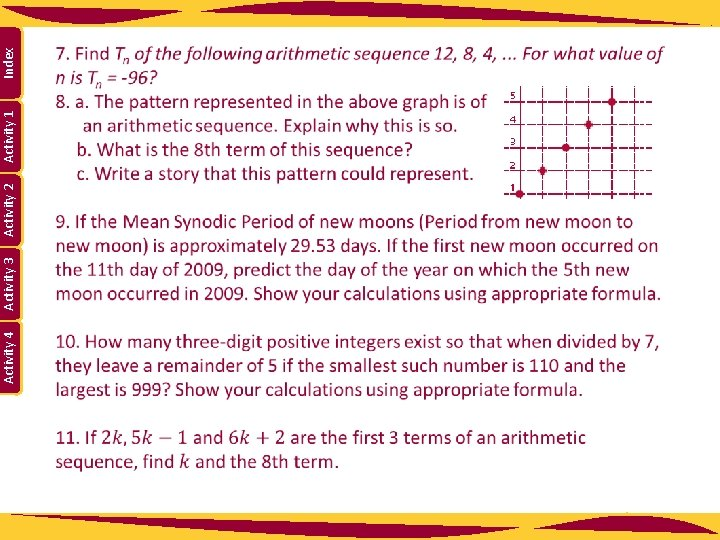Activity 4 Activity 3 Activity 2 Activity 1 Index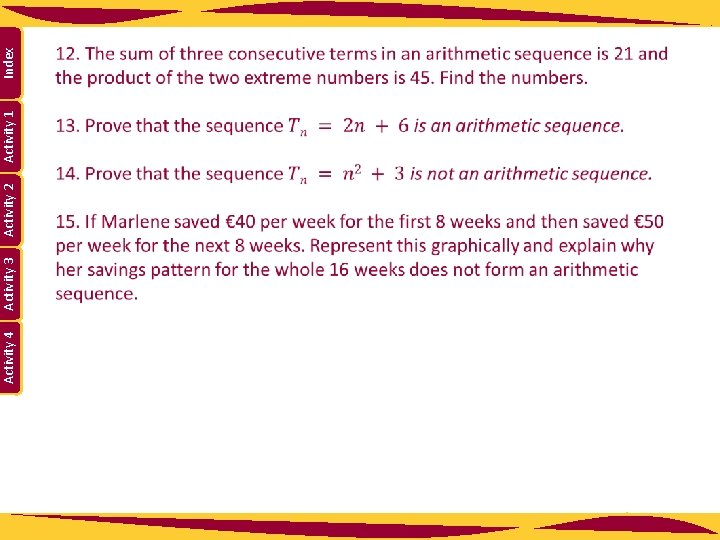Activity 4 Activity 3 Activity 2 Activity 1 Index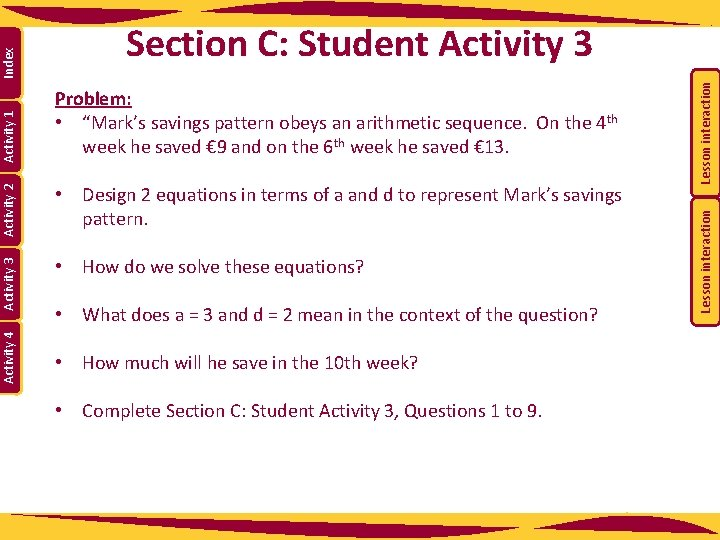• What does a = 3 and d = 2 mean in the context of the question? • How much will he save in the 10 th week? • Complete Section C: Student Activity 3, Questions 1 to 9. Lesson interaction • How do we solve these equations? Lesson interaction Index Activity 1 Activity 2 • Design 2 equations in terms of a and d to represent Mark’s savings pattern. Activity 4 Problem: • “Mark’s savings pattern obeys an arithmetic sequence. On the 4 th week he saved € 9 and on the 6 th week he saved € 13. Activity 3 Section C: Student Activity 3Index Activity 1 Activity 2 Activity 3 Activity 4 Section C: Student Activity 3 (Calculations must be shown in all cases. ) 1. The tenth term of an arithmetic sequence is 40 and the twentieth term is 30. Find the common difference and the first term. 2. A doctor asked a patient to reduce his medication by a regular number of tablets per week. The reduction plan begins one week after the visit to the doctor. If 10 weeks after the visit to the doctor the person is taking 40 tablets and 20 weeks after the visit the person is taking 30. Find the weekly reduction in his consumption of tablets. 3. Patricia is climbing up a large number of steps and to encourage her on her way, her friend started counting the number of steps she completes every minute. From then on she keeps a regular pace. After 2 minutes she has climbed 56 steps in total and after 8 minutes she has climbed 158 steps in total. a. Find the number of steps she climbs every minute once her friend started counting. b. Find the number of steps she had climbed before her friend started counting. If she had not maintained a regular pace, would you still have been able to use this method?Index Activity 1 Activity 2 Activity 3 Activity 4 4. If the 9 th floor of a building is 40 metres above the ground and the ground floor is 4 metres in height and each floor apart from the ground floor has equal height. Find the height of each floor. (Note the 1 st floor is the one above the ground floor etc. ) 5. On the first day of a month a baker receives a delivery of flour and starts using this flour at the beginning of the following day. He uses a regular amount of flour each day thereafter. At the end of the 4 th day of the month he has 1, 000 kg of flour remaining and at the end of the 12 th of the month 640 kg remain. How much flour does he use per day and how much flour did he have delivered? Use equations to represent this information and then solve the equations. 6. A woman has a starting salary of € 20, 000 and after the first year she gets an annual increase of € 2, 000 per year. Find her annual salary when she reaches retirement age, 40 years from when she started the job?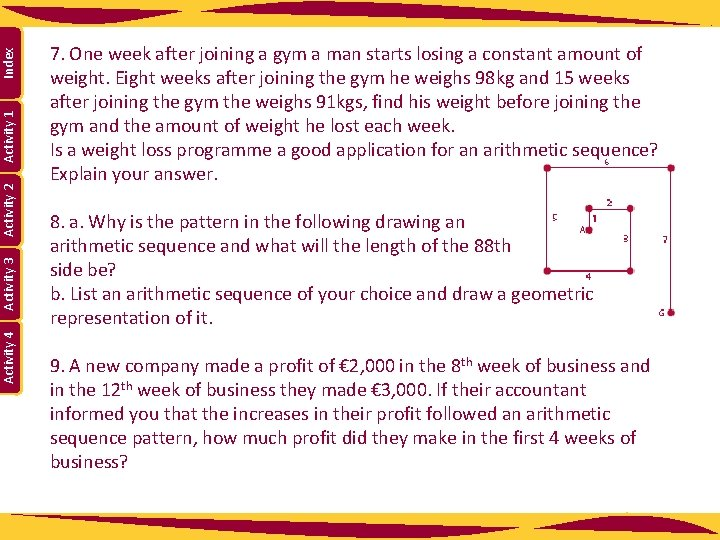Index Activity 1 Activity 2 Activity 3 Activity 4 7. One week after joining a gym a man starts losing a constant amount of weight. Eight weeks after joining the gym he weighs 98 kg and 15 weeks after joining the gym the weighs 91 kgs, find his weight before joining the gym and the amount of weight he lost each week. Is a weight loss programme a good application for an arithmetic sequence? Explain your answer. 8. a. Why is the pattern in the following drawing an arithmetic sequence and what will the length of the 88 th side be? b. List an arithmetic sequence of your choice and draw a geometric representation of it. 9. A new company made a profit of € 2, 000 in the 8 th week of business and in the 12 th week of business they made € 3, 000. If their accountant informed you that the increases in their profit followed an arithmetic sequence pattern, how much profit did they make in the first 4 weeks of business?Index Activity 1 Activity 2 Activity 3 Activity 4 Work in pairs and devise your own question(s) similar to the ones in this Student Activity. Then give the questions to the group next to you so that they can solve them.• A mobile phone supplier charges € 5 plus € 0. 10 per call. • What is the charge when the user makes 8 calls? • Which term of the sequence is this? • Does the cost of using this supplier form an arithmetic sequence? • Why do you say it forms an arithmetic sequence? • What value has a in this sequence? • What value has d in this sequence? • Which term in the sequence represents the 10 th call? • This is something you need to watch out for in questions. • Explain why it happened in this question? • Now attempt questions 10 -12 in Section C: Student Activity 3. Lesson interaction Index Activity 1 Activity 2 Activity 3 Activity 4 Solving Problems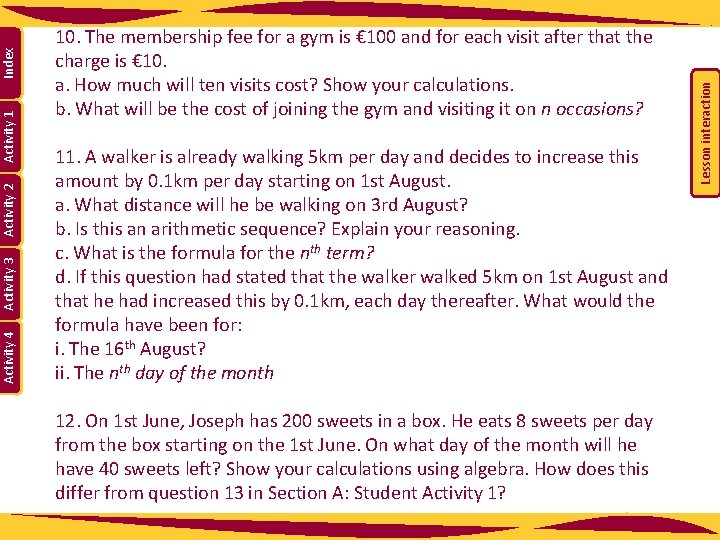11. A walker is already walking 5 km per day and decides to increase this amount by 0. 1 km per day starting on 1 st August. a. What distance will he be walking on 3 rd August? b. Is this an arithmetic sequence? Explain your reasoning. c. What is the formula for the nth term? d. If this question had stated that the walker walked 5 km on 1 st August and that he had increased this by 0. 1 km, each day thereafter. What would the formula have been for: i. The 16 th August? ii. The nth day of the month 12. On 1 st June, Joseph has 200 sweets in a box. He eats 8 sweets per day from the box starting on the 1 st June. On what day of the month will he have 40 sweets left? Show your calculations using algebra. How does this differ from question 13 in Section A: Student Activity 1? Lesson interaction Index Activity 1 Activity 2 Activity 3 Activity 4 10. The membership fee for a gym is € 100 and for each visit after that the charge is € 10. a. How much will ten visits cost? Show your calculations. b. What will be the cost of joining the gym and visiting it on n occasions?• See Section D: Student Activity 4 • There is a famous sequence called the Fibonacci Sequence. Lesson interaction • Explain your reason. Activity 3 Activity 1 • Are all sequences arithmetic? Activity 2 Index Section D: Student Activity 4Fibonacci was an Italian mathematician, who worked on this problem in the 13 th century. He studied how fast rabbits breed in ideal circumstances. He imagined a newly born pair of rabbits, one male and one female were placed in a field and made the following assumptions about the mating habits of rabbits: • Rabbits mate at exactly one month old and mate every month after that. • Rabbits always have litters of exactly one male and one female. • The gestation period for rabbits is exactly one month. (Not true) • Rabbits never die. (Not true) Lesson interaction Index Activity 1 Activity 2 Activity 3 Activity 4 Section D: Student Activity 4• Can you give examples of other sequences that are not arithmetic? • There is a famous sequence called the Fibonacci Sequence. • Is this an arithmetic sequence? Lesson interaction Activity 1 • See Section D: Student Activity 4 • Explain your reason. Activity 2 • Are all sequences arithmetic? Activity 3 Index Section D: Student Activity 4Lesson interaction Index Activity 1 Activity 2 Activity 3 Activity 4 1 How many pairs will there be after 1 month? Explain. 2 How many pairs will there be after 2 months? Explain. 3 How many pairs will there be after 3 months? Explain. 4 How many pairs will there be after 4 months? Explain. 5 How many pairs will there be after 5 months? Explain. 6 How many pairs will there be after 6 months? Explain. 7 Can you see a pattern developing?Lesson interaction Index Activity 1 Activity 2 Activity 3 Activity 4 Now, I want you all to try the interactive quiz called “Arithmetic Sequence Quiz” on the Student’s CD.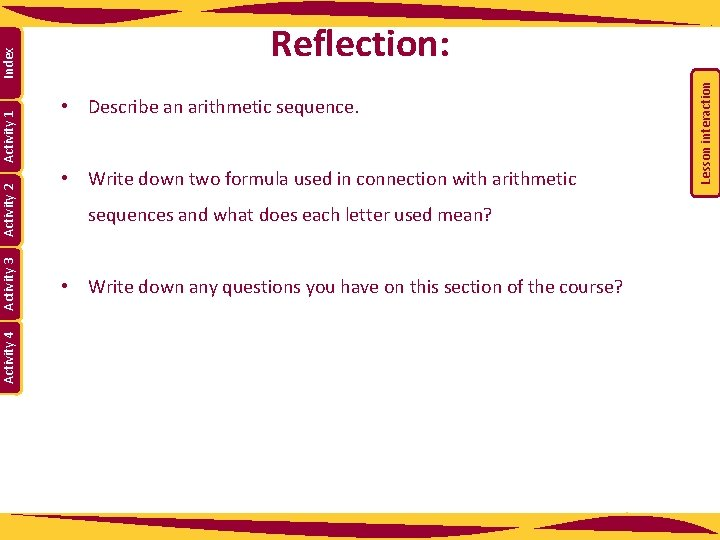• Describe an arithmetic sequence. • Write down two formula used in connection with arithmetic sequences and what does each letter used mean? • Write down any questions you have on this section of the course? Lesson interaction Index Activity 1 Activity 2 Activity 3 Activity 4 Reflection: